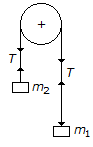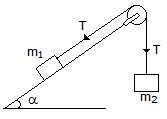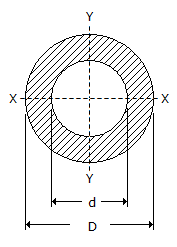Last updated: August 14, 2020

1. A body will begin to move down an inclined plane if the angle of inclination of the plane is __________ the angle of friction.
2. A body of weight W is required to move up on rough inclined plane whose angle of inclination with the horizontal is α. The effort applied parallel to the plane is given by(where μ = tanφ = Coefficient of friction between the plane and the body.)
3. The frequency of oscillation of a torsional pendulum is
4. When a particle moves along a circular path with uniform velocity, there will be no tangential acceleration.
5. If a number of forces are acting at a point, their resultant will be inclined at an angle θ with the horizontal, such that
6. The total time taken by a projectile to reach maximum height and to return back to the ground, is known as time of flight.
7. If the masses of both the bodies, as shown in the below figure, are reduced to 50 percent, then tension in the string will be8. In the shown figure, if the angle of inclination of the plane is increased, then acceleration of the system will9. the two equal forces at right angles acting at a point. The value of force R acting along their bisector and in opposite direction is10. Static friction is always __________ dynamic friction.
11. The force which acts along the radius of a circle and directed away from the centre of the circle is called centrifugal force.
12. A redundant frame is also called __________ frame.
13. Moment of inertia of a hollow circular section, as shown in the below figure about X-axis, is>
14. The maximum frictional force, which comes into play, when a body just begins to slide over the surface of the other body, is known as
15. If two bodies having masses m1 and m2 (m1>m2) have equal kinetic energies, the momentum of body having mass m1 is __________ the momentum of body having mass m2.
16. The centre of gravity of an equilateral triangle with each side a, is __________ from any of the three sides.
17. Two like parallel forces are acting at a distance of 24 mm apart and their resultant is 20 N. It the line of action of the resultant is 6 mm from any given force, the two forces are
18. A resultant force is a single force which produces the same effect as produced by all the given forces acting on a body.
19. The triangle law of forces states that if two forces acting simultaneously on a particle, be represented in magnitude and direction by the two sides of a triangle taken in order, then their resultant may be represented in magnitude and direction by the third side of a triangle, taken in opposite order.
20. The path of the projectile is a parabola.
Engineering Mechanics MCQ 2
You got {{userScore}} out of {{maxScore}} correct
{{title}}
{{image}}
{{content}}